• 输出利用先序遍历创建的二叉树的层次遍历序列 1000(ms) 10000(kb) 3377 / 7530 利用先序递归遍历算法创建二叉树并输出该二叉树的层次遍历序列。先序递归遍历建立二叉树的方法为：按照先序递归遍历的思想将对...
输出利用先序遍历创建的二叉树的层次遍历序列

1000(ms)

10000(kb)

3377 / 7530

利用先序递归遍历算法创建二叉树并输出该二叉树的层次遍历序列。先序递归遍历建立二叉树的方法为：按照先序递归遍历的思想将对二叉树结点的抽象访问具体化为根据接收的数据决定是否产生该结点从而实现创建该二叉树的二叉链表存储结构。约定二叉树结点数据为单个大写英文字符。当接收的数据是字符"#"时表示该结点不需要创建，否则创建该结点。最后再输出创建完成的二叉树的层次遍历序列。需要注意输入数据序列中的"#"字符和非"#"字符的序列及个数关系，这会最终决定创建的二叉树的形态。

输入

输入为接受键盘输入的由大写英文字符和"#"字符构成的一个字符串（用于创建对应的二叉树）。

输出

每个用例用一行出该用例对应的二叉树的层次遍历序列。

样例输入

A##

ABC####

AB##C##

ABCD###EF##G##H##

A##B##

样例输出

A

ABC

ABC

ABHCEDFG

A

import java.io.BufferedInputStream;
import java.io.ByteArrayInputStream;
import java.io.IOException;
import java.io.InputStream;
import java.util.ArrayList;
import java.util.Queue;
import java.util.Scanner;

public class Main {
static Queue<Node> nodes = new LinkedList<>();
static class Node{
int data;
Node left;
Node right;
public Node(){}

public int getData() {
return data;
}

public void setData(int data) {
this.data = data;
}

public Node getLeft() {
return left;
}

public void setLeft(Node left) {
this.left = left;
}

public Node getRight() {
return right;
}

public void setRight(Node right) {
this.right = right;
}
}
public static void createNode(Node node,BufferedInputStream bif){
try {
int ch ;
if(ch=='#'){
node=null;
return;
}else{
node.setData(ch);
node.setLeft(new Node());
node.setRight(new Node());
createNode(node.left,bif);
createNode(node.right,bif);
}
}else{
return;
}
}catch (IOException e){

}
}
return;
}
while(!nodes.isEmpty()){
Node newNode = nodes.poll();
if(newNode.data!=0) {
System.out.print((char) newNode.data);
}
if(newNode.left !=null) {
}
}

}
public static void main(String[] args){
BufferedInputStream is = new BufferedInputStream(System.in);
Node n = new Node();
createNode(n,is);
print(n);
}

}



展开全文• 下面三种序列可以唯一的构造唯一的一棵...层次遍历序列和中序遍历序列构造二叉树 #include<stdio.h> #include<stdlib.h> #include<string.h> #include<math.h> #define MaxSize 10 typ...
下面三种序列可以唯一的构造唯一的一棵二叉树：

前序序列和中序序列构造二叉树

后序序列和中序序列构造二叉树

层次遍历序列和中序遍历序列构造二叉树

#include<stdio.h>
#include<stdlib.h>
#include<string.h>
#include<math.h>
#define MaxSize 10
typedef struct BTNode{
char data;
struct BTNode *lchild,*rchild;
}BTNode,*BiTree;

//根据前序序列，中序序列建造一棵二叉树
//参数含义：pre[]前序序列  in[]中序序列
//			L1  R1用于指定pre[]的搜索区域
//			L2  R2用于指定in[]的搜索区域
BiTree createBiTree(char pre[],char in[],int L1,int R1,int L2,int R2){
if(L1>R1){
return NULL;//递归出口
}
BTNode *s =(BTNode*)malloc(sizeof(BTNode));
s->lchild =NULL;
s->rchild =NULL;
s->data=pre[L1];
int i;
//根据中序序列的特性，元素左边的元素属于该节点的左孩子序列，右边则是右孩子序列
for(i=L2;i<=R2;i++){
if(pre[L1]==in[i]) break;//在中序序列找到该元素
}
s->lchild=createBiTree(pre,in,L1+1,L1+i-L2,L2,i-1);//递归
s->rchild=createBiTree(pre,in,L1+i-L2+1,R1,i+1,R2);

return s;
}
//根据后序中序建立二叉树
BiTree createBiTree2(char post[],char in[],int L1,int R1,int L2,int R2){
if(L1>R1){
return NULL;//递归出口
}
BTNode *s =(BTNode*)malloc(sizeof(BTNode));
s->lchild =NULL;
s->rchild =NULL;
s->data=post[R1];//后序序列最后一个节点为根节点
int i;
//根据中序序列的特性，元素左边的元素属于该节点的左孩子序列，右边则是右孩子序列
for(i=L2;i<=R2;i++){
if(post[R1]==in[i]) break;//在中序序列找到该元素
}
//递归 面试官：为什么要减去L2? 答：递归传下去的是整个数组，需要根据参数找到递归出口,-L2是缩小查找范围
s->lchild=createBiTree2(post,in,L1,L1+i-L2-1,L2,i-1);
s->rchild=createBiTree2(post,in,L1+i-L2,R1-1,i+1,R2);

return s;
}
//该函数用于在中序序列中找到key,返回其位置
//参数含义：in[]：中序序列  key:所要查找元素 L,R:查找范围
int serach(char in[],char key,int L,int R){
for(int i=L;i<=R;i++){
if(in[i]==key) return i;
}
return -1;
}

//因为在中序序列元素的左面的元素在层次遍历序列中并不连续，所以需要用子序列函数将并不连续的序列连续起来
//为什么要连续起来？因为需要连续起来才能和中序序列构造出二叉树
//思路：在中序序列里查找到一个范围，从这个范围里遍历元素，在层次遍历序列里找该元素
//参数含义：sublevel[]：存放子序列数组  level[]：层次遍历序列  in[]:中序序列 n:level层次序列长度 L,R:查找范围
void getSubLevel(char sublevel[],char level[],char in[],int n,int L,int R){
int k=0;
for(int i=0;i<n;i++){
if(serach(in,level[i],L,R)!=-1)
sublevel[k++]=level[i];
}
}
//由中序序列和层次遍历序列构造二叉树
BiTree createBiTree3(char level[],char in[],int n,int L,int R){
if(L>R) return NULL;
BTNode *s =(BTNode*)malloc(sizeof(BTNode));
s->lchild =NULL;
s->rchild =NULL;
s->data=level;
int i=serach(in,level,L,R);
//找到i便可以确定左右子树答大小
int LN=i-L; char LLevel[LN];
int RN=R-i; char RLevel[RN];
getSubLevel(LLevel,level,in,n,L,i-1);//得到子序列
getSubLevel(RLevel,level,in,n,i+1,R);

s->lchild=createBiTree3(LLevel,in,LN,L,i-1);//用子序列递归
s->rchild=createBiTree3(RLevel,in,RN,i+1,R);
return s;
}
void visit(BiTree T) {
printf("%c  ",T->data);
}
//前序遍历
void PreOrder(BiTree T){
if(T!=NULL){
visit(T);//根
PreOrder(T->lchild);//左
PreOrder(T->rchild);//右
}
}
//中序遍历
void InOrder(BiTree T){
if(T!=NULL){
InOrder(T->lchild);
visit(T);
InOrder(T->rchild);
}
}
//后序遍历
void PostOrder(BiTree T){
if(T!=NULL){
PostOrder(T->lchild);
PostOrder(T->rchild);
visit(T);
}
}
//层次遍历
void level(BiTree T){
if(T!=NULL){
int front=0;
int rear=0;
BiTree queue[MaxSize];
BiTree p;
p=T;
rear=(rear+1)%MaxSize;
queue[rear]=p;
while(rear!=front){
front=(front+1)%MaxSize;
p=queue[front];
visit(p);
if(p->lchild!=NULL){
rear=(rear+1)%MaxSize;
queue[rear]=p->lchild;
}
if(p->rchild!=NULL){
rear=(rear+1)%MaxSize;
queue[rear]=p->rchild;
}

}
}
}
int main(){
char c[]="ABDECFGH";//前序序列
char d[]="DBEACGFH";//中序序列
char p[]="DEBGHFCA";//后序序列
char l[]="ABCDEFGH";//层次遍历序列
BTNode *r=createBiTree(c,d,0,7,0,7);
BTNode *r2=createBiTree2(p,d,0,7,0,7);
BTNode *r3=createBiTree3(l,d,8,0,7);
printf("前序序列为：");
PreOrder(r);
printf("\n");
printf("后序序列为：");
PostOrder(r2);
printf("\n");
printf("中序序列为：");
InOrder(r3);
printf("\n");
printf("层次遍历为：");
level(r3);
printf("\n");

} 

代码运行截图：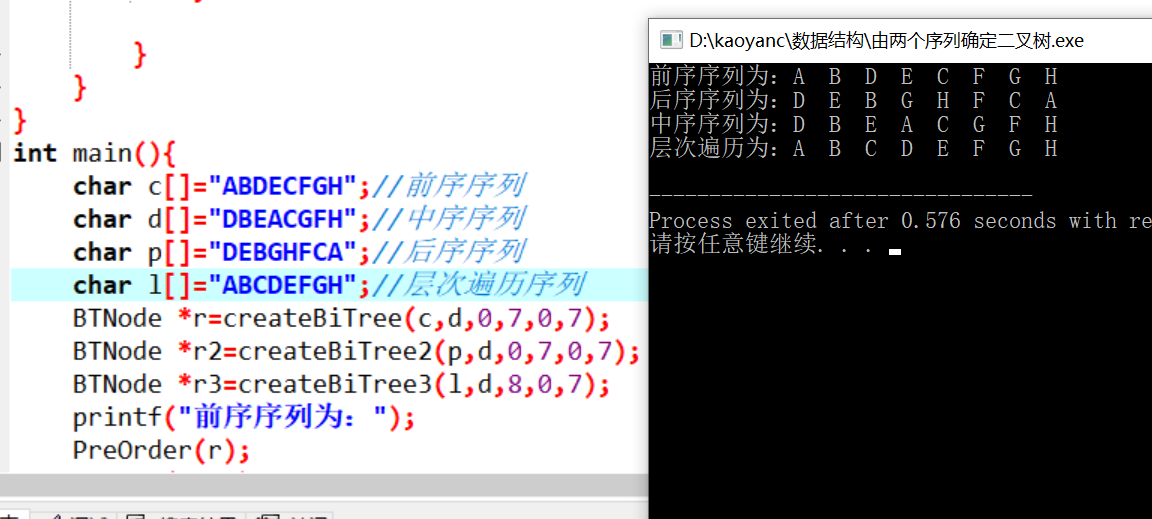展开全文• 只不过层次遍历序列比较特殊，它的结点、左子树、右子树不能一下看出。 中心思想： 通过中序序列找到根结点索引（根结点之前为左子树，之后为右子树），再从层次序列分别找到对应的左、右子树（要注意按照层次序列的...
前面有写过已知先序和中序的建树过程，其实大概原理都差不多。
只不过层次遍历序列比较特殊，它的结点、左子树、右子树不能一下看出。
中心思想：
通过中序序列找到根结点索引（根结点之前为左子树，之后为右子树），再从层次序列分别找到对应的左、右子树（要注意按照层次序列的顺序去找，代码中双重循环处体现，原因是：要保持递归的第一个参数是层次遍历序列，第二个参数是中序遍历序列）。#include <iostream>
#include <string.h>
#define type char
using namespace std;
typedef struct tree
{
type data;  //结点数据
tree *l,*r; //左结点、右结点
}btree;

btree *create(type *layer,type *mid,int len)
{
if(len<=0)return NULL;
btree *p=new btree;
p->data=*layer; //存根结点
int i,index;
for(i=0;i<len;i++)
if(*layer==mid[i])
{	index=i;break;  //根结点索引
}
int j,k=0;
type t1;
//从层次遍历序列找到左子树存入一个新的数组
for(i=0;i<len;i++)	 //此行与下一行顺序不可调换
for(j=0;j<index;j++)
{	if(layer[i]==mid[j])
t1[k++]=layer[i];
}
t1[k]='\0'; //不写没影响
p->l=create(t1,mid,k); //创建左子树
j=index+1,k=0;
type t2;
//从层次遍历序列找到右子树存入一个新的数组
for(i=0;i<len;i++)
for(j=index+1;j<len;j++)
{	if(layer[i]==mid[j])
t2[k++]=layer[i];
}
t2[k]='\0'; //不写没影响
p->r=create(t2,mid+index+1,k); //创建右子树
return p;
}

int main()
{
type *layer=new type;
type *mid=new type;
cin>>layer>>mid;  //输入层次、中序遍历序列
btree *root=create(layer,mid,strlen(mid));
return 0;
}



展开全文• 输出利用先序遍历创建的二叉树的层次遍历序列 这种可以不用层次遍历算法，可以用递归给每层都标记然后计算二叉树的深度，利用深度创建一个二维数组，再利用标记和递归算法将每层的数据存到二维数组相应的位子，然后...
输出利用先序遍历创建的二叉树的层次遍历序列
这种可以不用层次遍历算法，可以用递归给每层都标记然后计算二叉树的深度，利用深度创建一个二维数组，再利用标记和递归算法将每层的数据存到二维数组相应的位子，然后再输出。具体代码实现如下：
首先利用先序遍历创建二叉树：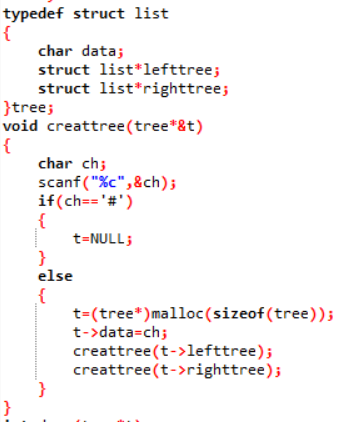然后再编写查找二叉树深度的程序：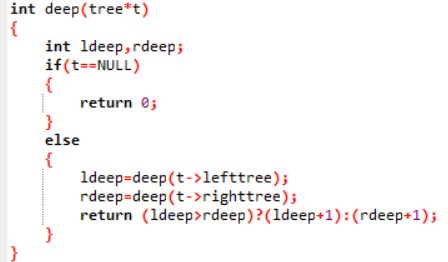然后再根据二叉树的深度创建一个二维数组，在编写一个算法将每层的数存到二维数组相应的位子算法如下：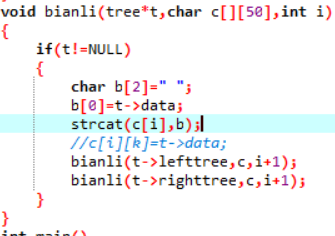然后编写主函数：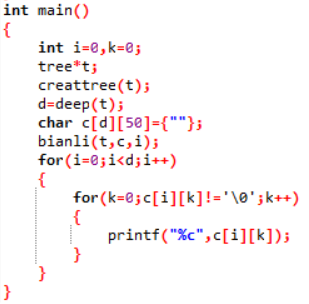程序的运行结果如下：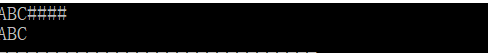展开全文• 1020. Tree Traversals (25) 时间限制 400 ms 内存限制 65536 kB ...代码长度限制 16000 B 判题程序 Standard 作者 CHEN, Yue Suppose that all the keys in a binary tree
• #include #include #include #include char s1,s2,s3,s4;... //printf("二叉树的中序序列和层次遍历序列相同\n"); return 0; } 转载于:https://www.cnblogs.com/-xss/p/6011924.html
• 层次遍历的同时，用中序序列求出子树的下标范围，并标记在子树根结点上。利用左右孩子为空的特殊情况，一层一层构造，直至叶子结点。 代码如下，思路详见注释： #include&lt;stdio.h&gt;#include&lt;...
• 代码】 #include <stdio.h> #include <stdlib.h> #include <math.h> #include <ctype.h> #include <string.h> #define maxSize 100 typedef char ElementType; typedef ...
• 层次遍历；由前序序列、中序序列重构二叉树；由后序序列、中序序列重构二叉树；# -*- coding: utf-8 -*-# @Time: 2019-04-15 18:35# @Author: chenclass NodeTree:def __init__(self, root=None, lchild=Non...java
• printf("二叉树的层次遍历结果为:"); LevelOrder(T); printf("\n"); return 0; } void init(QUEUE & Q) { Q.front = (pNode)malloc(sizeof(Node)); if(NULL == Q.front) { printf("动态分配内存失败\n");...c++ 数据结构算法
• 二叉树的层次遍历 ...按加入空树信息的先序遍历序列建立二叉树的二叉链表代码提供如下： //先序遍历序列建立二叉链表 public static BtNode createBiTree() throws IOException{ char c=(char) System.in.
• T是二叉树树根指针，Levelorder函数输出给定二叉树的层次遍历序列，格式为一个空格跟着一个字符。 其中BinTree结构定义如下： typedef char ElemType; typedef struct BiTNode { ElemType data; struct ...
• 二叉树的层次遍历 编写程序，要求实现 （1）按先序遍历序列建立二叉树的二叉链表；（2）按层次遍历二叉树。...按加入空树信息的先序遍历序列建立二叉树的二叉链表代码提供如下： //先序遍历序列建立二叉链表 void Cc++ 数据结构
• 2021年02月12日 周五 天气晴 【不悲叹过去，不荒废现在，不惧怕未来】 ...左”，代码上和前序遍历只是略有区别。 而中序遍历得到的二叉树不唯一，不能保证反序列化的二叉树为原来的树，如下图所示。 图源队列 dfs
• 树的遍历时间限制400 ms内存限制65536 kB代码长度限制8000 B判题程序Standard作者陈越给定一棵二叉树的后序遍历和中序遍历，请你输出其层序遍历序列。这里假设键值都是互不相等的正整数。输入格式：输入第一行给...递归
• 6-9 先序建树层次遍历 （25 分） 编写程序，要求实现（1）按先序遍历序列建立二叉树的二叉链表；...按加入空树信息的先序遍历序列建立二叉树的二叉链表代码提供如下： //先序遍历序列建立二叉链表 public static B...
• 输出这棵二叉树的层次遍历序列。 ##样例输入 ABC##DE#G##F### #样例输出 ABCDEFG 代码实现: #include<stdio.h> #include<stdlin=b.h> #define MAX 100 typedef struct node{ char data; ...
• c++代码，能够根据前序序列、中序序列生成二叉树；能够一步生成，也可以一步步自动生成。 设计前序遍历，中序遍历，后序遍历，层次遍历
• importjava.util.ArrayList;importjava.util.List;importjava.util.Stack;/*** 总结了三种非递归遍历二叉树*@author85060**/public classTraverseBinaryTree {//用来装遍历序列的一个list，展示的时候用pr...
• 问题：给一棵二叉树的层序遍历序列和中序遍历序列，求这棵二叉树的先序遍历序列和后序遍历序列。 分析：层次序列第一个元素肯定是根结点了，那么在读入后序元素过程中只需要确定它是根节点的左子树还是右子树问题了...
• 按加入空树信息的先序遍历序列建立二叉树的二叉链表代码提供如下： //先序遍历序列建立二叉链表 public static BtNode createBiTree() throws IOException{ char c=(char) System.in.read(); .
• 呜呜呜笨蛋把前序遍历和层次遍历搞错了？ 题目不难，细心即可。 根据前序遍历的第一个元素取中序遍历中找将树分成两个部分，不断的递归就好啦，详细的看代码代码 /** * Definition for a binary tree node. * ...算法
• 数据结构学过有一段时间了，太长时间没有写...二叉树的建立①：（用扩展先序遍历序列创建二叉树） #include #include #include #include using namespace std; typedef struct Node{ char data; struct Node *数据结构 扩展
• 由于之前王老师讲了怎么通过递归的方式利用 遍历序列的输入顺序构建 二叉树 一、思想基于递归遍历 例如 先序遍历 1 2 4 0 0 5 6 0 0 7 0 0 3 0 0 -1 (0表示当前结点为空，-1表示输入结束) 二、构建二叉树 1.先判断...
• 编写程序，要求实现（1）按先序遍历序列建立二叉树的二叉链表；（2）按层次遍历二叉树。 C++： 构成二叉链表的结点类代码如下： ...按加入空树信息的先序遍历序列建立二叉树的二叉链表代码提供如下： //先
• 二叉树的序列化与反序列化完整代码 题目：leetcode297. 二叉树的序列化与反序列序列化是将一个数据结构或者对象转换为连续的比特位的操作，进而可以将转换后的数据存储在一个文件或者内存中，同时也可以通过网络...
• 输出二叉树的层次遍历序列。 输入样例 ab#c##d## 输出样例 abdc 链二叉树结点代码 typedef struct treeNode { char data; struct treeNode* lchild, * rchild; }treeNode, * BiTree; 先序遍历创建二叉树 主要...
• 数据结构系列（1）树——二叉树的构造、层次遍历、节点个数、树的高度题目分析解题步骤解题代码 题目分析 当我们通过前序序列和中序序列构造出二叉树后，再进行层次遍历： 解题步骤 我们首先通过二叉树的前序...数据结构 算法...